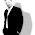## Sunday, August 25, 2019

### Techie Post - Nested If in Excel for converting month to a number

This post is more of a reminder for me, but may help someone else in a similar situation.  Every year in August when I need to create the new user accounts for the students starting in September at school, I need the student date of births to be in the format DDMMYY so that my custom powershell script can create the accounts in Active Directory

Sounds simple, but alas our School Information Management System exports the data in a weird format, so I am always having to modify and fix the exported data in excel before I can import the completed csv file.

Most can be accomplished using the excellent left, mid and right formulas, but the date (more specifically, the month) always causes me issues.

So, where I a have a cell where the month is "Jan", or "Feb", I need it to be "01" for Jan, "02" for Feb and so on.

So.... assuming cell F2 is the cell with the month in it, the following nested If formula will do the job

=IF(F2="Jan","01",IF(F2="Feb","02",IF(F2="Mar","03",IF(F2="Apr","04",IF(F2="May","05",IF(F2="Jun","06",IF(F2="Jul","07",IF(F2="Aug","08",IF(F2="Sep","09",IF(F2="Oct","10",IF(F2="Nov","11",IF(F2="Dec","12",))))))))))))

Once I have the month as a number, I can easily combine the 3 numbers making up the day, month and year (ddmmyy) in to a cell for my script to accept.

I'm sure there's a better way of doing it, but hey ho...  This does the job for now.

1.2.